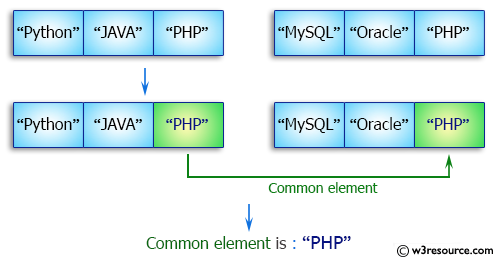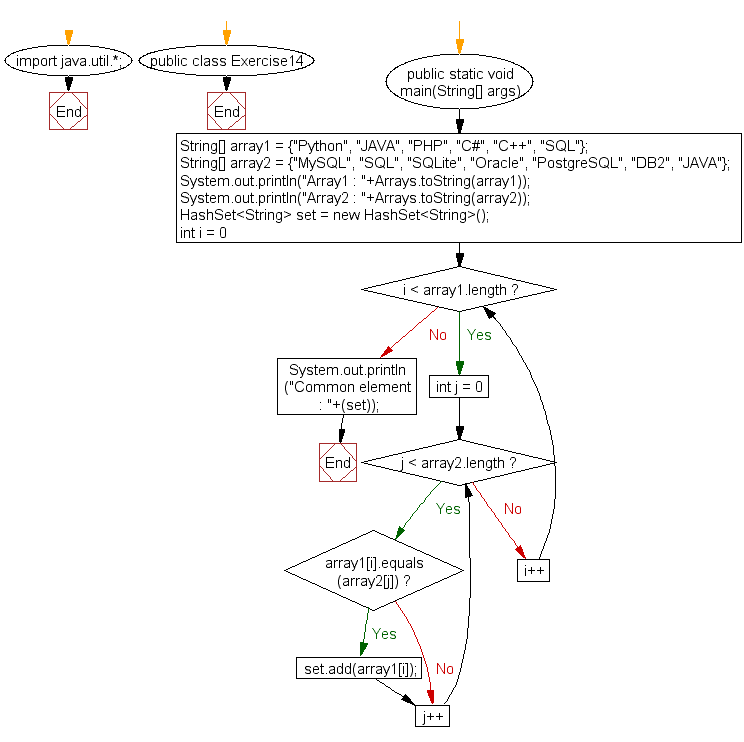﻿ Java exercises: Find the common elements between two arrays - w3resource# Java Array Exercises: Find the common elements between two arrays

## Java Array: Exercise-14 with Solution

Write a Java program to find the common elements between two arrays (string values).

Pictorial Presentation:Sample Solution:

Java Code:

``````import java.util.*;
public class Exercise14 {
public static void main(String[] args)
{
String[] array1 = {"Python", "JAVA", "PHP", "C#", "C++", "SQL"};

String[] array2 = {"MySQL", "SQL", "SQLite", "Oracle", "PostgreSQL", "DB2", "JAVA"};

System.out.println("Array1 : "+Arrays.toString(array1));
System.out.println("Array2 : "+Arrays.toString(array2));

HashSet<String> set = new HashSet<String>();

for (int i = 0; i < array1.length; i++)
{
for (int j = 0; j < array2.length; j++)
{
if(array1[i].equals(array2[j]))
{
}
}
}

System.out.println("Common element : "+(set));     //OUTPUT : [THREE, FOUR, FIVE]
}
}
```
```

Sample Output:

```Array1 : [Python, JAVA, PHP, C#, C++, SQL]
Array2 : [MySQL, SQL, SQLite, Oracle, PostgreSQL, DB2, JAVA]
Common element is : [JAVA, SQL]
```

Flowchart:Visualize Java code execution (Python Tutor):

Java Code Editor:

Improve this sample solution and post your code through Disqus

What is the difficulty level of this exercise?

Test your Programming skills with w3resource's quiz.

﻿

## Java: Tips of the Day

countOccurrences

Counts the occurrences of a value in an array.

Use Arrays.stream().filter().count() to count total number of values that equals the specified value.

```public static long countOccurrences(int[] numbers, int value) {
return Arrays.stream(numbers)
.filter(number -> number == value)
.count();
}
```

Ref: https://bit.ly/3kCAgLb